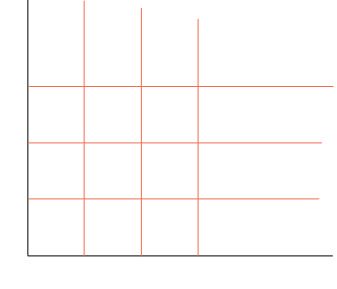SEARCH HOMEMath Central Quandaries & QueriesQuestion from Larry, a student: Change 1meter(to 3rd power) to centimetre(to 3rd power). This is a metric conversion problemHi Larry,

I can get you started.

1 meter is 100 centimetres. Imagine you have a large piece of paper which is 1 meter by 1 meter. Draw a grid on the paper that is 1 centimetre by 1 centimetre.How many 1 cm by 1 cm squares do you have. This is the number of $1 cm^2$ in $1 m^2.$

PennyMath Central is supported by the University of Regina and The Pacific Institute for the Mathematical Sciences.